# Tag Archives | SigmaXL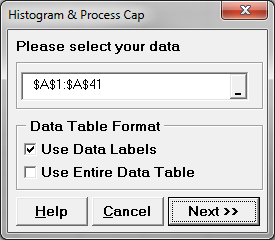## Process Capability with SigmaXL

Process Capability Process capability measures how well the process performs to meet given specified outcome. It indicates the conformance of a process to meet given requirements or specifications. Capability analysis helps to better understand the performance of the process with respect to meeting customer’s specifications and identify the process improvement opportunities. Process Capability Analysis Steps […]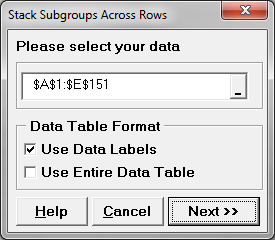## Attribute MSA with SigmaXL

Use SigmaXL to Implement an Attribute MSA Data File: “Attribute MSA” tab in “Sample Data.xlsx” (an example in the AIAG MSA Reference Manual, 3rd Edition). Step 1: Reorganize the original data into four new columns (i.e., Appraiser, Assessed Result, Part, and Reference). Select the entire range of the original data (“Part”, “Reference”, “Appraiser A”, “Appraiser […]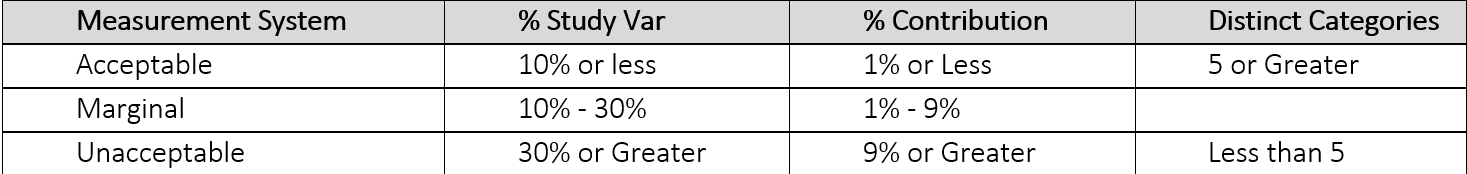## Variable Gage R&R with SigmaXL

Variable Gage R&R Variable Gage Repeatability & Reproducibility (Gage R&R) is a method used to analyze the variability of a measurement system by partitioning the variation of the measurements using ANOVA (Analysis of Variance). Whenever something is measured repeatedly or by different people or processes, the results of the measurements will vary. Variation comes from […]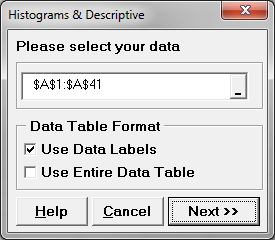## Histogram Rendering with SigmaXL

What is a Histogram? A histogram is a graphical tool to present the distribution of the data. These plots are used to better understand how values occur in a given set of data. The X axis   represents the possible values of the variable and the Y axis represents the frequency of the value occurring. This graphical […]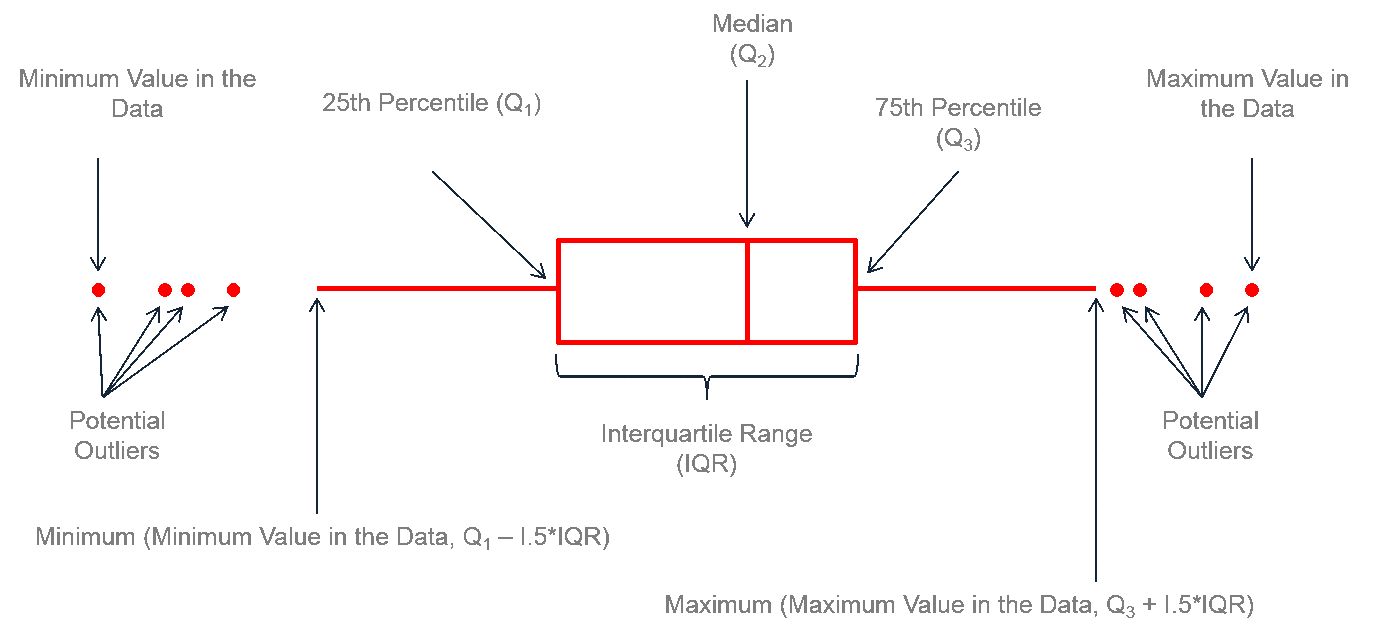## Box Plot with SigmaXL

What is a Box Plot? In statistics, graphical analysis is a method to visualize the quantitative data. Graphical analysis is used to discover structure and patterns in the data. The presence of which may explain or suggest reasons for additional analysis or consideration. A complete statistical analysis includes both quantitative analysis and graphical analysis. When […]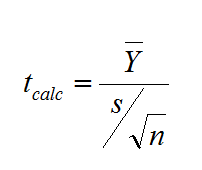## One Sample t Test with SigmaXL

What is a One Sample t Test? A One sample t test is a hypothesis test to study whether there is a statistically significant difference between a population mean and a specified value. Where: What is a t-Test? In statistics, a t-test is a hypothesis test in which the test statistic follows a Student’s t […]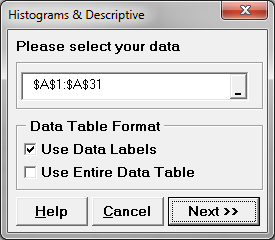## Central Limit Theorem with SigmaXL

Central Limit Theorem The Central Limit Theorem is one of the fundamental theorems of probability theory. It states a condition under which the mean of a large number of independent and identically-distributed random variables, each of which has a finite mean and variance, would be approximately normally distributed. Let us assume Y1, Y2 . . […]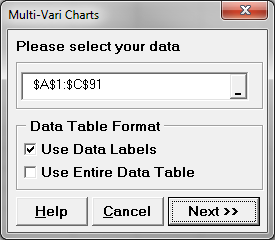## Multi Vari Analysis with SigmaXL

Multi-Vari Analysis Multi Vari Analysis is a graphic-driven method to analyze the effects of categorical inputs on a continuous output. It studies how the variation in the output changes across different inputs and helps us quantitatively determine the major source of variability in the output. Multi Vari charts are used to visualize the source of […]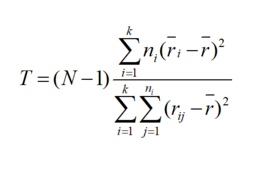## Kruskal Wallis with SigmaXL

Kruskal–Wallis One-Way Analysis of Variance The Kruskal Wallis one-way analysis of variance is a statistical hypothesis test to compare the medians among more than two groups. It is an extension of Mann–Whitney test. While the Mann–Whitney test allows us to compare the samples of two populations, the Kruskal–Wallis test allows us to compare the samples […]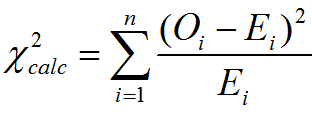## Chi Square Test with SigmaXL

Chi Square (Contingency Tables) We have looked at hypothesis tests to analyze the proportion of one population vs. a specified value, and the proportions of two populations, but what do we do if we want to analyze more than two populations? A chi-square test is a hypothesis test in which the sampling distribution of the […]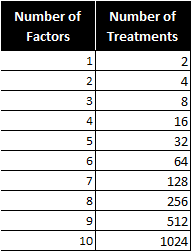## Fractional Factorial Designs with SigmaXL

What Are Fractional Factorial Experiments? In simple terms, a fractional factorial experiment is a subset of a full factorial experiment. Fractional factorials use fewer treatment combinations and runs. Fractional factorials are less able to determine effects because of fewer degrees of freedom available to evaluate higher order interactions. Fractional factorials can be used to screen [...]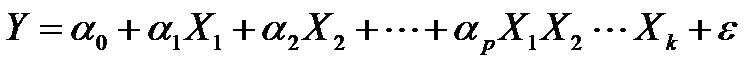## Full Factorial DOE with SigmaXL

What is a Full Factorial DOE? In a full factorial experiment, all of the possible combinations of factors and levels are created and tested. For example, for two-level design (i.e.each factor has two levels) with k factors, there are 2k possible scenarios or treatments. Two factors, each with two levels, we have 22 = 4 treatments [...]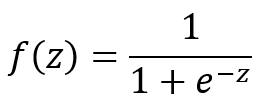## Logistic Regression with SigmaXL

What is Logistic Regression? Logistic regression is a statistical method to predict the probability of an event occurring by fitting the data to a logistic curve using logistic function. The regression analysis used for predicting the outcome of a categorical dependent variable, based on one or more predictor variables. The logistic function used to model [...]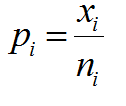## P Chart with SigmaXL

What is a P Chart? The P chart plots the percentage of defectives in one subgroup as a data point. It considers the situation when the subgroup size of inspected units is not constant. The underlying distribution of the P-chart is binomial distribution. P Chart Equations Data Point:         Center Line:   [...]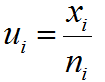## U Chart with SigmaXL

What is a U Chart? The U chart is a type of control chart used to monitor discrete (count) data where the sample size is greater than one, typically the average number of defects per unit. Defect vs. Defective Remember the difference between defect and defective? A defect of a unit is the unit’s characteristic [...]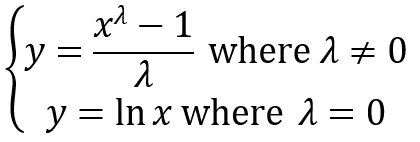## Box Cox Transformation with SigmaXL

Box Cox Transformation Data transforms are usually applied so that the data appear to more closely meet assumptions of a statistical inference model to be applied or to improve the interpret-ability or appearance of graphs. Power transformation is a class of transformation functions that raise the response to some power. For example, a square root [...]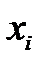## IR Chart with SigmaXL

What is an IR Chart? The IR chart (also called individual-moving range chart or I-MR chart) is a popular control chart for continuous data with subgroup size equal to one. The I chart plots an individual observation as a data point. The MR chart plots the absolute value of the difference between two consecutive observations [...]